GeeksforGeeks App
Open AppBrowser
Continue

# Merge two sorted arrays

Given two sorted arrays, the task is to merge them in a sorted manner.
Examples:

Input: arr1[] = { 1, 3, 4, 5}, arr2[] = {2, 4, 6, 8}
Output: arr3[] = {1, 2, 3, 4, 4, 5, 6, 8}

Input: arr1[] = { 5, 8, 9}, arr2[] = {4, 7, 8}
Output: arr3[] = {4, 5, 7, 8, 8, 9}

Naive Approach:

It is the brute force method to do the same. Take all the elements of arr1 and arr2 in arr3. Then simply sort the arr3.

The implementation of above approach is:

## C++

 `// C++ program to merge two sorted arrays/``#include``using` `namespace` `std;` `void` `mergeArrays(``int` `arr1[], ``int` `arr2[], ``int` `n1,``                            ``int` `n2, ``int` `arr3[])``{``    ``int` `i = 0, j = 0, k = 0;``      ``// traverse the arr1 and insert its element in arr3``      ``while``(i < n1){``      ``arr3[k++] = arr1[i++];``    ``}``      ` `      ``// now traverse arr2 and insert in arr3``      ``while``(j < n2){``      ``arr3[k++] = arr2[j++];``    ``}``      ` `      ``// sort the whole array arr3``      ``sort(arr3, arr3+n1+n2);``}` `// Driver code``int` `main()``{``    ``int` `arr1[] = {1, 3, 5, 7};``    ``int` `n1 = ``sizeof``(arr1) / ``sizeof``(arr1);` `    ``int` `arr2[] = {2, 4, 6, 8};``    ``int` `n2 = ``sizeof``(arr2) / ``sizeof``(arr2);` `    ``int` `arr3[n1+n2];``    ``mergeArrays(arr1, arr2, n1, n2, arr3);` `    ``cout << ``"Array after merging"` `<

## Java

 `// Java program to merge two sorted arrays/``import` `java.util.*;` `public` `class` `GFG {``    ``// Driver code``    ``public` `static` `void` `main(String[] args) {``        ``int` `arr1[] = {``1``, ``3``, ``5``, ``7``};``        ``int` `n1 = arr1.length;` `        ``int` `arr2[] = {``2``, ``4``, ``6``, ``8``};``        ``int` `n2 = arr2.length;` `        ``int` `arr3[] = ``new` `int``[n1 + n2];``        ``mergeArrays(arr1, arr2, n1, n2, arr3);` `        ``System.out.println(``"Array after merging"``);``        ``for` `(``int` `i=``0``; i < n1+n2; i++)``            ``System.out.print(arr3[i] + ``" "``);``            ` `    ``}``    ` `    ``public` `static` `void` `mergeArrays(``int``[] arr1, ``int``[] arr2, ``int` `n1, ``int` `n2, ``int``[] arr3){``        ``int` `i = ``0``; ``        ``int` `j = ``0``; ``        ``int` `k = ``0``; ``        ` `        ``// traverse the arr1 and insert its element in arr3``        ``while``(i < n1){  ``         ``arr3[k++] = arr1[i++];  ``        ``}  ``        ` `        ``// now traverse arr2 and insert in arr3``        ``while``(j < n2){  ``         ``arr3[k++] = arr2[j++];  ``        ``}  ``        ` `        ``// sort the whole array arr3``        ``Arrays.sort(arr3);  ``    ``}``}` `// This code is contributed by Tapesh(tapeshdua420)`

## Python3

 `# Python program to merge two sorted arrays/``def` `mergeArrays(arr1, arr2, n1, n2, arr3):``    ``i ``=` `0``    ``j ``=` `0``    ``k ``=` `0` `    ``# traverse the arr1 and insert its element in arr3``    ``while``(i < n1):``        ``arr3[k] ``=` `arr1[i]``        ``k ``+``=` `1``        ``i ``+``=` `1` `    ``# now traverse arr2 and insert in arr3``    ``while``(j < n2):``        ``arr3[k] ``=` `arr2[j]``        ``k ``+``=` `1``        ``j ``+``=` `1` `    ``# sort the whole array arr3``    ``arr3.sort()`  `# Driver code``if` `__name__ ``=``=` `'__main__'``:``    ``arr1 ``=` `[``1``, ``3``, ``5``, ``7``]``    ``n1 ``=` `len``(arr1)` `    ``arr2 ``=` `[``2``, ``4``, ``6``, ``8``]``    ``n2 ``=` `len``(arr2)` `    ``arr3 ``=` `[``0` `for` `i ``in` `range``(n1``+``n2)]``    ``mergeArrays(arr1, arr2, n1, n2, arr3)` `    ``print``(``"Array after merging"``)``    ``for` `i ``in` `range``(n1 ``+` `n2):``        ``print``(arr3[i], end``=``" "``)` `# This code is contributed by Tapesh(tapeshdua420)`

## C#

 `// C# program to merge two sorted arrays``using` `System;``using` `System.Collections.Generic;` `public` `class` `GFG``{``    ``public` `static` `void` `mergeArrays(``int``[] arr1, ``int``[] arr2, ``int` `n1, ``int` `n2, ``int``[] arr3) {``        ``int` `i = 0;``        ``int` `j = 0;``        ``int` `k = 0;` `        ``// traverse the arr1 and insert its element in arr3``        ``while` `(i < n1) {``            ``arr3[k++] = arr1[i++];``        ``}` `        ``// now traverse arr2 and insert in arr3``        ``while` `(j < n2) {``            ``arr3[k++] = arr2[j++];``        ``}` `        ``// sort the whole array arr3``        ``Array.Sort(arr3);``    ``}` `    ``public` `static` `void` `Main(``string``[] args)``    ``{``        ``int` `[]arr1 = ``new` `int``[] {1, 3, 5, 7};``        ``int` `n1 = arr1.Length;` `        ``int` `[]arr2 = ``new` `int``[] {2, 4, 6, 8};``        ``int` `n2 = arr2.Length;` `        ``int` `[]arr3 = ``new` `int``[n1 + n2];``        ``mergeArrays(arr1, arr2, n1, n2, arr3);` `        ``Console.WriteLine(``"Array after merging"``);``        ``for` `(``int` `i = 0; i < n1 + n2; i++)``            ``Console.Write(arr3[i] + ``" "``);``    ``}``}` `// This code is contributed by ajaymakavana.`

## Javascript

 `  ``// JavaScript program to merge two sorted arrays/``  ``function` `mergeArrays(arr1, arr2, n1, n2, arr3) {``      ``var` `i = 0, j = 0, k = 0;``      ` `      ``// traverse the arr1 and insert its element in arr3``      ``while` `(i < n1) {``          ``arr3[k++] = arr1[i++];``      ``}` `      ``// now traverse arr2 and insert in arr3``      ``while` `(j < n2) {``          ``arr3[k++] = arr2[j++];``      ``}` `      ``// sort the whole array arr3``      ``arr3.sort();``  ``}``  ``var` `arr1 = [1, 3, 5, 7];``  ``var` `n1 = arr1.length;``  ``var` `arr2 = [2, 4, 6, 8];``  ``var` `n2 = arr2.length;``  ``var` `arr3 = ``new` `Array(n1 + n2);``  ``mergeArrays(arr1, arr2, n1, n2, arr3);` `  ``console.log(``"Array after merging"``);``  ``for` `(``var` `i = 0; i < n1 + n2; i++)``      ``process.stdout.write(arr3[i] + ``" "``);` `// This code is contributed by tapeshdua420.`

Output

```Array after merging
1 2 3 4 5 6 7 8 ```

Time Complexity : O((m+n) log(m+n)) , the whole size of arr3 is m+n
Auxiliary Space: O(1), No extra space is used

Method 2 (O(n1 * n2) Time and O(n1+n2) Extra Space)

1. Create an array arr3[] of size n1 + n2.
2. Copy all n1 elements of arr1[] to arr3[]
3. Traverse arr2[] and one by one insert elements (like insertion sort) of arr3[] to arr1[]. This step take O(n1 * n2) time.

We have discussed implementation of above method in Merge two sorted arrays with O(1) extra space

Method 3 (O(n1 + n2) Time and O(n1 + n2) Extra Space)
The idea is to use Merge function of Merge sort

1. Create an array arr3[] of size n1 + n2.
2. Simultaneously traverse arr1[] and arr2[].
• Pick smaller of current elements in arr1[] and arr2[], copy this smaller element to next position in arr3[] and move ahead in arr3[] and the array whose element is picked.
3. If there are remaining elements in arr1[] or arr2[], copy them also in arr3[].

Below is the illustration of the above approach:

Take two sorted arrays Array1 and Array2 and an Empty Array3 . Take three pointers  ‘i’, ‘j’, and ‘k’ for comparisons, here ‘i’ pointer points towards 0th index of Array1, similarly ‘j’ and ‘k’ pointer point towards 0th index of Array2 and Array3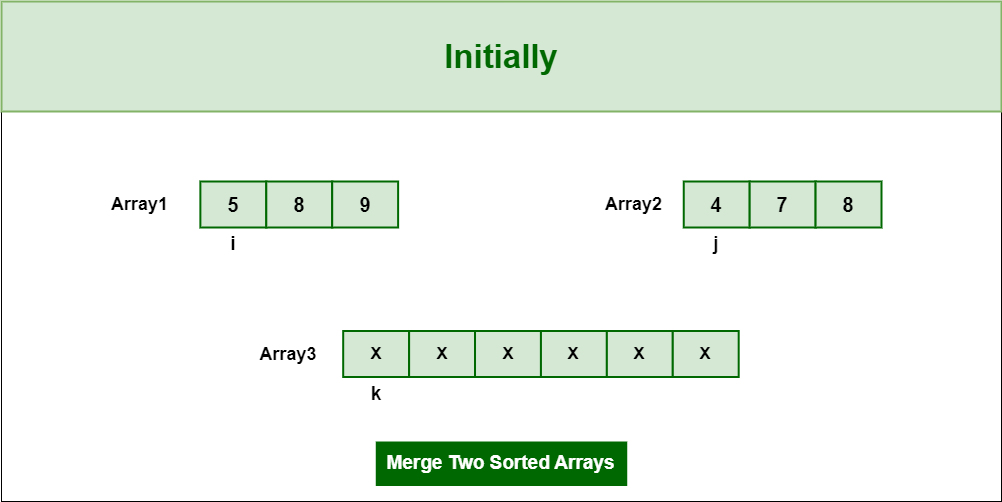Initial Arrays

Step 1: Pick Smaller element which is 4 and insert in into Array3 and update the pointer ‘j ‘and ‘k’ after comparing ‘i’ and ‘j’.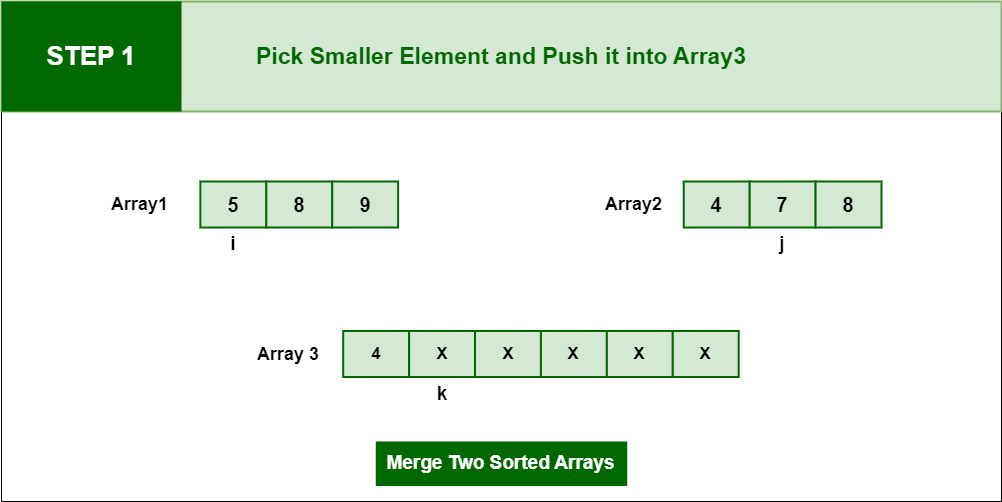Pick Smaller element which is 4

Step 2: Pick next smaller element which is 5 and insert in into Array3 and update the pointer ‘i’ and ‘k’ after comparing ‘i’ and ‘j’.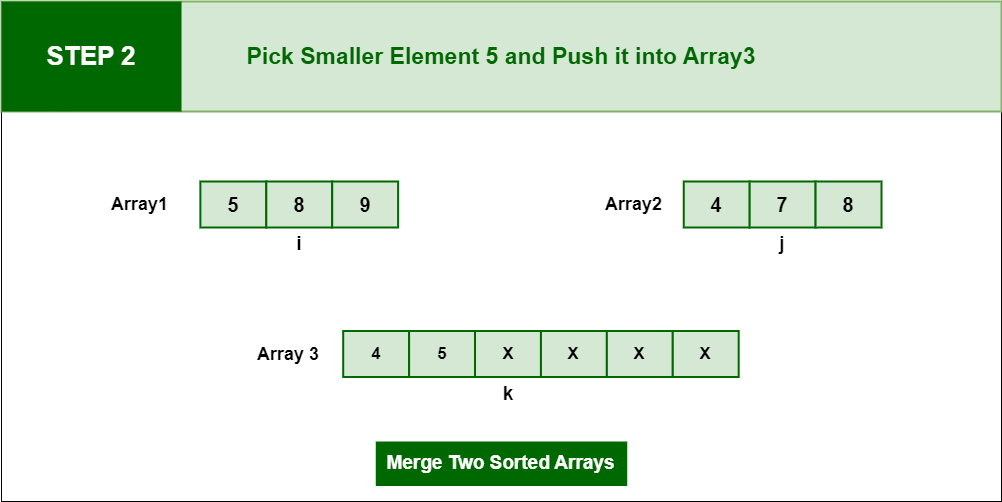Pick next smaller element which is 5

Step 3: Pick next smaller element which is 7 and insert in into Array3 and update the pointer ‘j’ and ‘k ‘after comparing ‘i’ and ‘j’.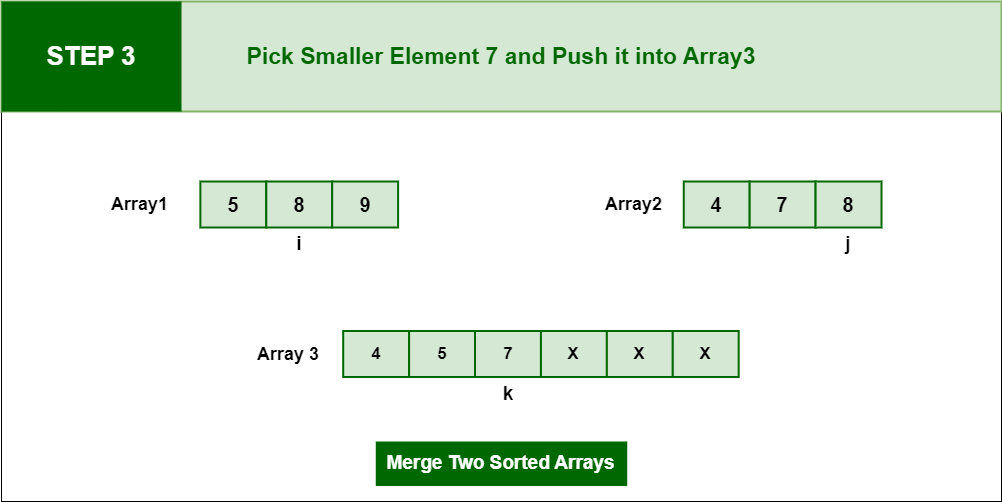Pick next smaller element which is 7

Step 4: when ‘j’ pointer meets the length of Array2 then first while loop breaks and second while loop copies all elements from arr1 to arr3.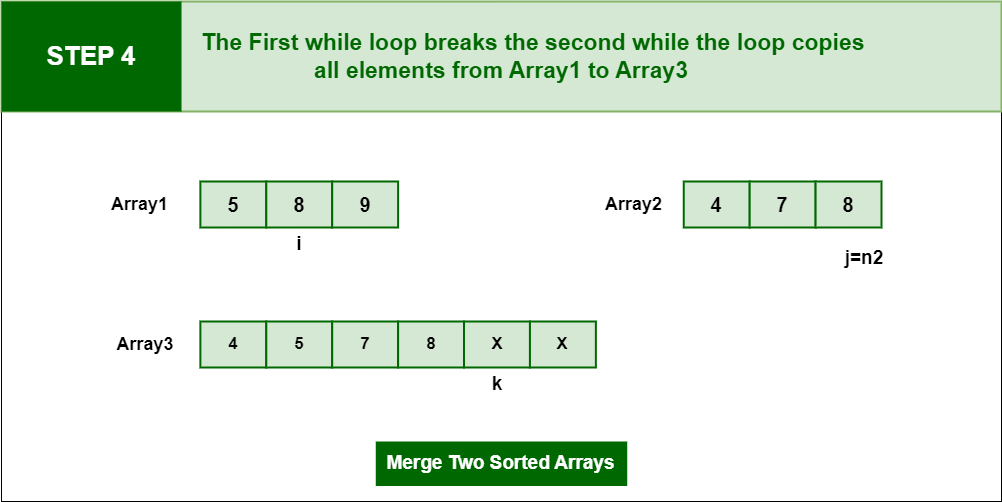second while loop copies all elements from arr1 to arr3.

Step 5:  Pick adjacent element from Array1 and insert in into Array3 and update the pointer i and k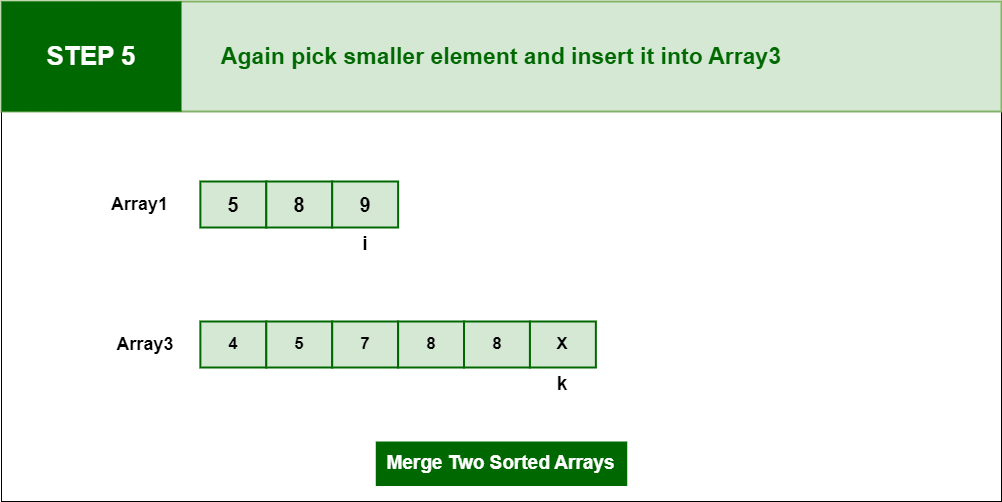Pick adjacent element from Array1 and insert in into Array3

Step 6:     Pick remaining element from Array1 and insert in into Array3, when i pointer meets the length of Array1 that means k = n1+n2 and at last we have merge sorted Array3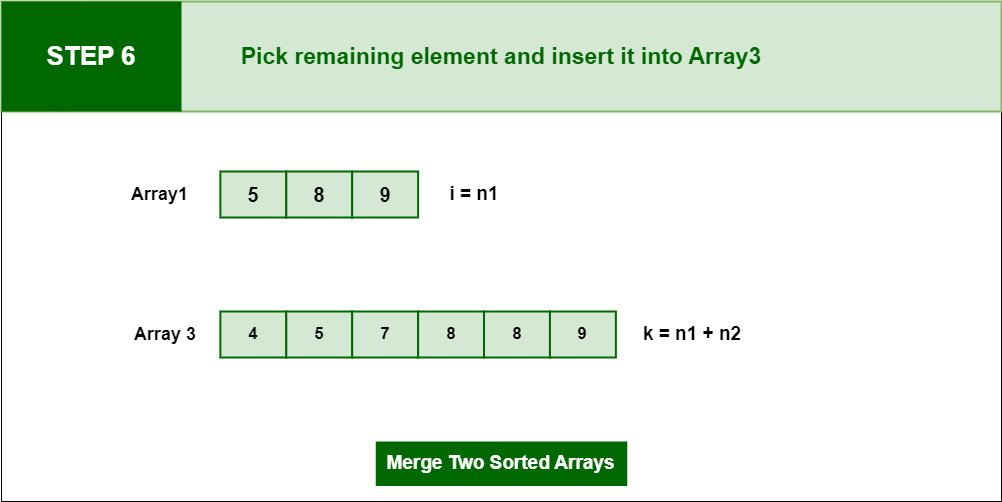ick remaining element from Array1 and insert in into Array3,

Below is the implementation of the above approach:

## C++

 `// C++ program to merge two sorted arrays/``#include``using` `namespace` `std;` `// Merge arr1[0..n1-1] and arr2[0..n2-1] into``// arr3[0..n1+n2-1]``void` `mergeArrays(``int` `arr1[], ``int` `arr2[], ``int` `n1,``                             ``int` `n2, ``int` `arr3[])``{``    ``int` `i = 0, j = 0, k = 0;` `    ``// Traverse both array``    ``while` `(i

## Java

 `// Java program to merge two sorted arrays``import` `java.util.*;``import` `java.lang.*;``import` `java.io.*;` `class` `MergeTwoSorted``{``    ``// Merge arr1[0..n1-1] and arr2[0..n2-1]``    ``// into arr3[0..n1+n2-1]``    ``public` `static` `void` `mergeArrays(``int``[] arr1, ``int``[] arr2, ``int` `n1,``                                ``int` `n2, ``int``[] arr3)``    ``{``        ``int` `i = ``0``, j = ``0``, k = ``0``;``    ` `        ``// Traverse both array``        ``while` `(i

## Python 3

 `# Python program to merge``# two sorted arrays` `# Merge arr1[0..n1-1] and``# arr2[0..n2-1] into``# arr3[0..n1+n2-1]``def` `mergeArrays(arr1, arr2, n1, n2):``    ``arr3 ``=` `[``None``] ``*` `(n1 ``+` `n2)``    ``i ``=` `0``    ``j ``=` `0``    ``k ``=` `0` `    ``# Traverse both array``    ``while` `i < n1 ``and` `j < n2:``    ` `        ``# Check if current element``        ``# of first array is smaller``        ``# than current element of``        ``# second array. If yes,``        ``# store first array element``        ``# and increment first array``        ``# index. Otherwise do same``        ``# with second array``        ``if` `arr1[i] < arr2[j]:``            ``arr3[k] ``=` `arr1[i]``            ``k ``=` `k ``+` `1``            ``i ``=` `i ``+` `1``        ``else``:``            ``arr3[k] ``=` `arr2[j]``            ``k ``=` `k ``+` `1``            ``j ``=` `j ``+` `1``    `  `    ``# Store remaining elements``    ``# of first array``    ``while` `i < n1:``        ``arr3[k] ``=` `arr1[i];``        ``k ``=` `k ``+` `1``        ``i ``=` `i ``+` `1` `    ``# Store remaining elements``    ``# of second array``    ``while` `j < n2:``        ``arr3[k] ``=` `arr2[j];``        ``k ``=` `k ``+` `1``        ``j ``=` `j ``+` `1``    ``print``(``"Array after merging"``)``    ``for` `i ``in` `range``(n1 ``+` `n2):``        ``print``(``str``(arr3[i]), end ``=` `" "``)` `# Driver code``arr1 ``=` `[``1``, ``3``, ``5``, ``7``]``n1 ``=` `len``(arr1)` `arr2 ``=` `[``2``, ``4``, ``6``, ``8``]``n2 ``=` `len``(arr2)``mergeArrays(arr1, arr2, n1, n2);` `# This code is contributed``# by ChitraNayal`

## C#

 `// C# program to merge``// two sorted arrays``using` `System;` `class` `GFG``{``    ``// Merge arr1[0..n1-1] and``    ``// arr2[0..n2-1] into``    ``// arr3[0..n1+n2-1]``    ``public` `static` `void` `mergeArrays(``int``[] arr1, ``int``[] arr2,``                                   ``int` `n1, ``int` `n2, ``int``[] arr3)``    ``{``        ``int` `i = 0, j = 0, k = 0;``    ` `        ``// Traverse both array``        ``while` `(i < n1 && j < n2)``        ``{``            ``// Check if current element``            ``// of first array is smaller``            ``// than current element``            ``// of second array. If yes,``            ``// store first array element``            ``// and increment first array``            ``// index. Otherwise do same``            ``// with second array``            ``if` `(arr1[i] < arr2[j])``                ``arr3[k++] = arr1[i++];``            ``else``                ``arr3[k++] = arr2[j++];``        ``}``    ` `        ``// Store remaining``        ``// elements of first array``        ``while` `(i < n1)``            ``arr3[k++] = arr1[i++];``    ` `        ``// Store remaining elements``        ``// of second array``        ``while` `(j < n2)``            ``arr3[k++] = arr2[j++];``    ``}``    ` `    ``// Driver code``    ``public` `static` `void` `Main()``    ``{``        ``int``[] arr1 = {1, 3, 5, 7};``        ``int` `n1 = arr1.Length;``    ` `        ``int``[] arr2 = {2, 4, 6, 8};``        ``int` `n2 = arr2.Length;``    ` `        ``int``[] arr3 = ``new` `int``[n1+n2];``    ` `        ``mergeArrays(arr1, arr2, n1, n2, arr3);``    ` `        ``Console.Write(``"Array after merging\n"``);``        ``for` `(``int` `i = 0; i < n1 + n2; i++)``            ``Console.Write(arr3[i] + ``" "``);``    ``}``}` `// This code is contributed``// by ChitraNayal`

## PHP

 ``

## Javascript

 ``

Output

```Array after merging
1 2 3 4 5 6 7 8 ```

Output:

```Array after merging
1 2 3 4 5 6 7 8```

Time Complexity : O(n1 + n2)
Auxiliary Space : O(n1 + n2)

Method 4: Using Maps (O(nlog(n) + mlog(m)) Time and O(N) Extra Space)

1. Insert elements of both arrays in a map as keys.
2. Print the keys of the map.

Below is the implementation of above approach.

## CPP

 `// C++ program to merge two sorted arrays``//using maps``#include``using` `namespace` `std;` `// Function to merge arrays``void` `mergeArrays(``int` `a[], ``int` `b[], ``int` `n, ``int` `m)``{``    ``// Declaring a map.``    ``// using map as a inbuilt tool``    ``// to store elements in sorted order.``    ``map<``int``, ``int``> mp;``    ` `    ``// Inserting values to a map.``    ``for``(``int` `i = 0; i < n; i++)mp[a[i]]++;``    ` `    ` `    ``for``(``int` `i = 0;i < m;i++)mp[b[i]]++;` `    ` `    ``// Printing keys of the map.``    ``for``(``auto` `j: mp)``    ``{``         ``for``(``int` `i=0; i

## Java

 `// Java program to merge two sorted arrays``//using maps``import` `java.io.*;``import` `java.util.*;` `class` `GFG {``    ` `    ``// Function to merge arrays``    ``static` `void` `mergeArrays(``int` `a[], ``int` `b[], ``int` `n, ``int` `m)``    ``{``      ` `        ``// Declaring a map.``        ``// using map as a inbuilt tool``        ``// to store elements in sorted order.``        ``Map mp = ``new` `TreeMap();``      ` `        ``// Inserting values to a map.``        ``for``(``int` `i = ``0``; i < n; i++)``        ``{``            ``mp.put(a[i], ``true``);``        ``}``        ``for``(``int` `i = ``0``;i < m;i++)``        ``{``            ``mp.put(b[i], ``true``);``        ``}``      ` `        ``// Printing keys of the map.``        ``for` `(Map.Entry me : mp.entrySet())``        ``{``            ``System.out.print(me.getKey() + ``" "``);``        ``}``    ``}``    ` `    ``// Driver Code``    ``public` `static` `void` `main (String[] args)``    ``{``        ``int` `a[] = {``1``, ``3``, ``5``, ``7``}, b[] = {``2``, ``4``, ``6``, ``8``};``        ``int` `size = a.length;``        ``int` `size1 = b.length;``        ` `        ``// Function call``        ``mergeArrays(a, b, size, size1);``    ``}``}` `// This code is contributed by rag2127`

## Python3

 `# Python program to merge two sorted arrays``# using maps``import` `bisect` `# Function to merge arrays``def` `mergeArrays(a, b, n, m):``    ``# Declaring a map.``    ``# using map as a inbuilt tool``    ``# to store elements in sorted order.``    ``mp``=``[]``    ` `    ``# Inserting values to a map.``    ``for` `i ``in` `range``(n):``        ``bisect.insort(mp, a[i])``        ` `    ``for` `i ``in` `range``(m):``        ``bisect.insort(mp, b[i])``    ` `    ``# Printing keys of the map.``    ``for` `i ``in` `mp:``        ``print``(i,end``=``' '``)``        ` `# Driver code``arr1 ``=` `[``1``, ``3``, ``5``, ``7``]``arr2 ``=` `[``2``, ``4``, ``6``, ``8``]``size ``=` `len``(arr1)``size1 ``=` `len``(arr2)` `# Function call``mergeArrays(arr1, arr2, size, size1)` `# This code is contributed by Pushpesh Raj`

## C#

 `// C# program to merge two sorted arrays``//using maps``using` `System;``using` `System.Collections.Generic;` `public` `class` `GFG {` `  ``// Function to merge arrays``  ``static` `void` `mergeArrays(``int` `[]a, ``int` `[]b, ``int` `n, ``int` `m)``  ``{` `    ``// Declaring a map.``    ``// using map as a inbuilt tool``    ``// to store elements in sorted order.``    ``SortedDictionary<``int``, Boolean> mp = ``new` `SortedDictionary<``int``, Boolean>();` `    ``// Inserting values to a map.``    ``for` `(``int` `i = 0; i < n; i++) {``      ``mp.Add(a[i], ``true``);``    ``}``    ``for` `(``int` `i = 0; i < m; i++) {``      ``mp.Add(b[i], ``true``);``    ``}` `    ``// Printing keys of the map.``    ``foreach` `(KeyValuePair<``int``, Boolean> me ``in` `mp) {``      ``Console.Write(me.Key + ``" "``);``    ``}``  ``}` `  ``// Driver Code``  ``public` `static` `void` `Main(String[] args) {``    ``int` `[]a = { 1, 3, 5, 7 };``    ``int` `[]b = { 2, 4, 6, 8 };``    ``int` `size = a.Length;``    ``int` `size1 = b.Length;` `    ``// Function call``    ``mergeArrays(a, b, size, size1);``  ``}``}` `// This code is contributed by gauravrajput1`

## Javascript

 ``

Output

`1 2 3 4 5 6 7 8 `

Time Complexity: O( nlog(n) + mlog(m) )
Auxiliary Space: O(N)